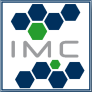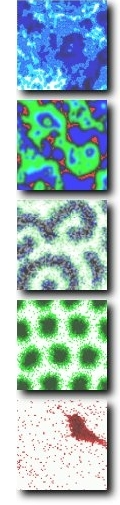Dept. for Innovative
Methods of Computing

### Studying Mathematical Biology in Dresden

###### General Information

The life sciences are rapidly turning from qualitative to quantitative sciences. To integrate the increasing amount of data in a systematic way development and application of mathematical models is required. The goal of Mathematical Biology is to gain an understanding of biological problems including genetics, evolution, cell and developmental biology by means of mathematical modeling.

The department Innovative Methods of Computing (head: Andreas Deutsch) within the Center for Information Services and High Performance Computing (ZIH) at Technische Universität Dresden conducts mathematical biology research and offers lectures/seminars providing a profound introduction to modern biomathematical concepts.

###### Aims/Prospects

• Interdisciplinary training in the rapidly developing area of mathematical biology
• Acquire profound knowledge of mathematical model structures and methods
• Conduct research (e.g. Master's or PhD project) within research groups at TU Dresden, MPI-CBG, MPI-PKS

###### Lectures

We offer the two-term lecture series Introduction to Mathematical Biology I - II. Course topics include deterministic, stochastic, statistical and cellular automaton models as well as the biological interpretation of the model analysis. Accompanying tutorials allow the active learning of important concepts. Each lecture part comprises 2+1 SWS. Lectures take place in the winter term.

###### Seminars

The Seminar in Mathematical Biology features on selected aspects of mathematical modelling of biological systems, e.g. development, epidemiology, pattern formation, regeneration, medical applications (e.g. cancer), and evolution. By means of talks and discussions, biological key questions and the application of suitable mathematical model classes are introduced. Seminar credits amount to 2 SWS. The seminar takes place in the summer term.